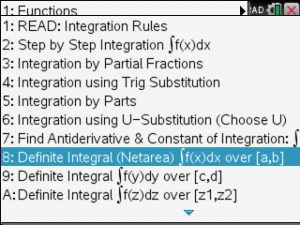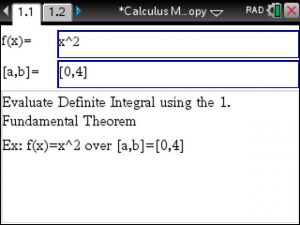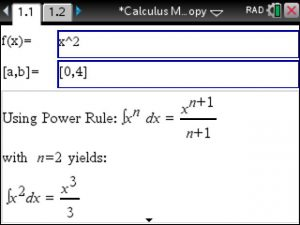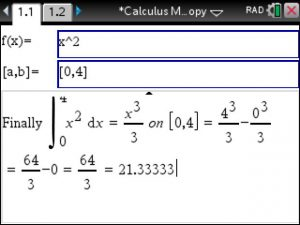# Ti-Nspire : Step by Step Definite Integrals

Computing definite Integrals using the 1. Fundamental Theorem of Calculus can be achieved using Calculus Made Easy at https://www.tinspireapps.com/?a=CME

Use option 8 in the INTEGRATION menuEnter function f(x) and bounds [a,b] as shown belowView the Integration steps, here, the Power Rule is applied.Lastly, plug the bounds into the above Antiderivative, subtract and that’s it!Posted on Categories calculus